Next:
Unitary Matrices Up: Lie Algebras

Elements of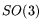act on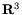, or equivalently on orthonormal bases of(frames).2 Butcan also be regarded as a set of transformations on the vector space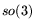, as we will see in a moment. Intuitively, the triple (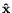,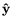,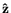) takes the place of the standard frame for.

General fact: for any Lie group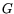, there is a homomorphism (also known as a representation) ofinto the group of non-singular linear transformations on the vector space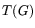, with kernel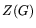, the center of.

Here's how it goes. For any group, we have the group of inner automorphism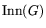and a homomorphism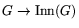defined by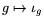, where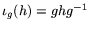. The kernel is. The automorphism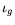is furthermore determined completely by its effects on any set of generators for.

Now taketo be a Lie group. Let's consider the effect ofon an infinitesimal'' generator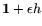, where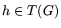.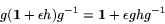Or in terms of derivatives, if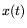is our prototypical smooth curve through 1, then the derivative of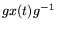at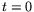is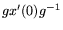. So the vector spaceis closed under the map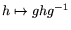. (Remember that bothandare sets of matrices.)is clearly contained in the kernel, and in fact:is the kernel.

For, this is rather intuitive. Suppose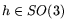is a rotation about the axis determined by vector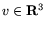. Then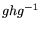is a rotation about the axis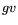: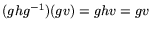. If we think of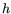as an infinitesimal rotation, then we see that the action ofongiven bylooks just like the action ofon.

Only in three dimensions do things work out so neatly.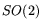is abelian, and the adjoint representation for abelian Lie groups is boring-- the trivial homomorphism. And the vector space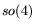has dimension 6, so the adjoint representation gives an imbedding ofin the group of non-singular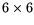matrices.Next: Unitary Matrices Up: Lie Algebras